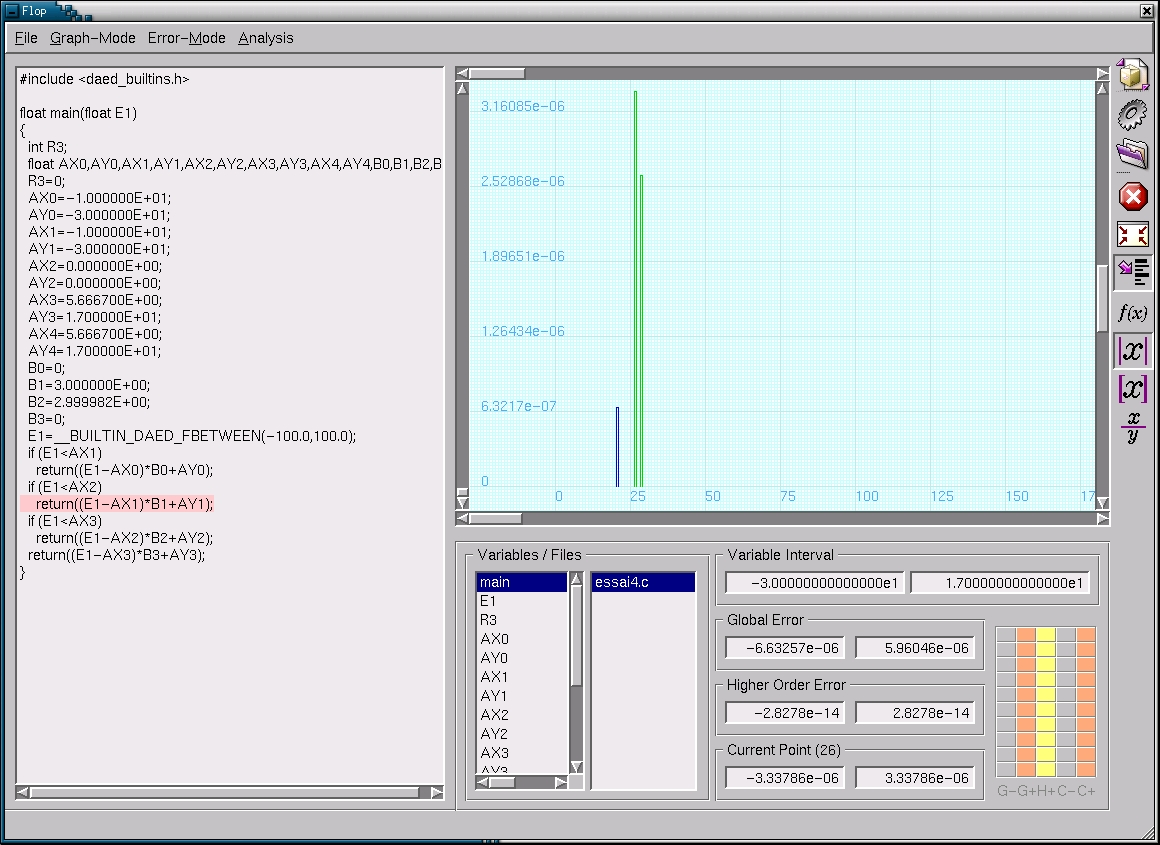FLUCTUAT  :   a Static Analyzer for Floating-point Operations

Content:

Context:
The manipulation of real numbers by computers is approximated by floating-point arithmetic, which uses a finite representation of numbers. This implies that a (small in general) rounding error may be committed  at each operation. Although this approximation is accurate enough for most applications, there are some cases where results become irrelevant because of the precision lost at some stages of the computation, even when the underlying numerical scheme is stable.
We aim at studying the propagation of rounding errors in floating-point computations, to detect automatically a possible catastrophic loss of precision, and its source. We are developping a static analyzer , intended to cope with real industrial problems, and we believe it is specially appropriate for critical instrumentation software.

Principle:
For example, let us examine a simple computation involving two values a=621.3 and b=1.287. For the sake of simplicity, we assume that a and b belong to a simplified set of floating-point numbers defined by the base b=10 and mantissa of size m=4.  In addition, let us assume that initial errors are attached to a and b,  and let us write

a=621.3+0.05 E1                 b = 1.287+ 0.0005 E2

to indicate that the value of the initial error on a (resp. b) is 0.05 (resp. 0.0005). a and b are called floating-point numbers with errors. Let us focus on the product a*b which exact result is a * b=799.6131. The same computation made with floating-point numbers with errors yields

a * b= 799.6131 + 0.06435 E1 + 0.31065 E2 + 0.000025 E1 E2

The difference between a * b and 621.35 * 1.2875 is 0.375025 and this error stems from the fact that the initial error on a (resp. b) was multiplied by b (resp a) and that a second order error corresponding to the multiplication of both errors was introduced. So, at the end of the computation, the contribution to the global error of the initial error on a is 0.06435 and corresponds to the coefficient attached to the formal variable E1. Similarly, the contribution to the global error of the initial error on b is denoted by the coefficient of E2. The contribution of the second order error due to  the initial errors on both a and b is given by the term 0.000025 E1 E2 which we rather write 0.000025 E1.2 in the following. Finally, the number 799.6131 has too many digits to be representable in our floating-point number system. Since IEEE Standard 754 ensures that elementary operations are correctly rounded, we may claim that the floating-point number computed by our machine is 799.6 and that a new error term 0.0131 E* is introduced by the multiplication. To sum up, we have

a * b = 799.6 + 0.06435 E1 + 0.31065 E2 +  0.000025E12 + 0.0131 E*

At first sight, one could believe that the precision of the computation mainly depends on the initial error on a since it is 100 times larger than the one on b. However, as shown by the Figure below, the above result indicates that the final error is mainly due to the initial error on b. Hence, to improve the precision of the final result, one should first try to increase the precision on b (whenever it is possible).The Fluctuat Analyzer:
As shown in the screenshot, the main window of the analyzer displays the code of the program being analyzed, the list of identifiers occurring in the abstract environment at the end of the analysis and a graph representation of the abstract value related to the selected identifier in the list.  Scrollbars on the sides of the graph window are used to do various kinds of zooms.

The graph represents the error series of a variable id and thus shows the contribution of the operations to the global error on id. The operations are identified with their program point which is displayed on the X-axis.

The bars indicate the maximum of the absolute values of the interval bounds. This enables to assert the correctness of the code, when the errors have a small magnitude. This kind of graph is well suited to identify some numerical errors, but other kinds of errors, like cancellations, are more easily detected by graphs showing relative errors.  Different types of graphs can be drawn by clicking the adequate button in the right-hand side toolbar.  The graph and program code frames are connected in the graphical user interface in such a way that clicking on a column of the graph makes the code frame emphasizes the related program block and conversely. This enables the user to easily identify which piece of code mainly contributes to the error
on the selected variable. Another interesting feature is that different grains of program points (like code lines or functions) can be selected by clicking the adequate button. Hence, for the analysis of a program made of many functions, the user may first identify which functions introduce the most important errors and next refine the result.Contacts:

Eric Goubault ,  email: goubault@aigle.saclay.cea.fr

Matthieu Martel , email: mmartel@cea.fr

Sylvie Putot, email: sputot@cea.fr